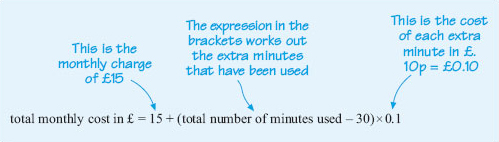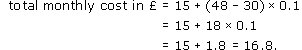Starting with maths: Patterns and formulas

Start this free course now. Just create an account and sign in. Enrol and complete the course for a free statement of participation or digital badge if available.

Free course

# Starting with maths: Patterns and formulas

One of the advantages of identifying the general features of a calculation and then describing it mathematically is that the formula can then be used in either a computer or a calculator program to work out many different calculations quickly and efficiently. Many utility suppliers (gas, water, electricity, telephone) have tariffs based on a fixed daily (or monthly or quarterly) charge and a further charge based on how much you have used during the billing period.

For example in 2005, a mobile phone network charged £15 per month for 30 minutes (or less) of phone calls. Extra calls above the 30 minutes were charged at 10p per minute.

Assuming that more than 30 minutes were used per month, the formula for the total monthly cost was given by

total monthly cost in £ = 15 + (total number of minutes used -30) × 0.1.

## Activity 6: Understanding the formula

Suppose that one month 75 minutes of phone calls were made. Explain how you would calculate the cost for the extra minutes (above the 30-minute allowance) and hence how to calculate the total cost for that month. Can you explain how the formula has been derived?

### Discussion

As 30 minutes are included in the £﻿15 charge, the number of minutes that are charged separately is

75 − 30 = 45.

Each extra minute costs £﻿0.1, so 45 extra minutes will cost 45 × £﻿0.1 = £﻿4.50.

So the total charge for that month is £﻿15 + £﻿4.50 = £19.50.

The formula says that the fixed charge is £﻿15, and then each minute in excess of the 30 minutes allowed costs 10p.Figure 10

## Activity 7: Phone bills

On this phone tariff, what would the monthly bill have been, if the total time for the calls were the following?

• (a) 48 minutes

• (b) 25 minutes

### Discussion

(a) Substituting 48 for the total number of minutes gives:Hence the bill for the month is £﻿16.80.

Note how this calculation is set out with a concluding sentence that answers the question precisely.

(b) The formula only applies if more than 30 minutes of calls are made, so it cannot be used in this case. This is an important step in using formulas – you do need to check that they apply in your particular situation before using them! Here, the charge is £﻿15 for up to 30 minutes of calls, so the charge for this month is £﻿15 overall.